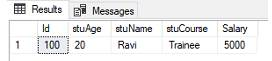# What is function in Database? explain some function like Sum(), Count(), Max(), Min(), AVG() with example. \$(function () { var favoriteStarId = "Q" + "93945"; var ExistsUrl = \$("#" + favoriteStarId).attr("ExistsUrl"); var favoriteData = { EntityId: "93945", EntityType: "Q", UserID: "0" } \$.ajax({ type: "POST", url: ExistsUrl, data: JSON.stringify(favoriteData), dataType: "json", contentType: 'application/json; charset=utf-8', success: function (response) { if (response.success == true) { if (response.isExist == true) { \$("#" + favoriteStarId).removeClass('qa-favorite-button'); \$("#" + favoriteStarId).addClass('qa-unfavorite-button'); } else { \$("#" + favoriteStarId).removeClass('qa-unfavorite-button'); \$("#" + favoriteStarId).addClass('qa-favorite-button'); } } } }); \$(".btn-fav").hover(function () { var classes = []; \$('.btn-fav').each(function () { classes.push(\$(this).attr('class')); }); if (classes == "btn-fav qa-favorite-button") { \$('.btn-fav').css("background-color", "#ffab0f"); } else { \$('.btn-fav').css("background-color", "#2f364a"); } \$('.btn-fav').css("background-position", "center bottom"); }, function () { var classes = []; \$('.btn-fav').each(function () { classes.push(\$(this).attr('class')); }); if (classes == "btn-fav qa-favorite-button") { \$('.btn-fav').css("background-color", "#2f364a"); } else { \$('.btn-fav').css("background-color", "#ffaf04"); } \$('.btn-fav').css("background-position", "center bottom"); }); })

46 views
asked 7 days ago in Database

What is function in Database? explain some function like Sum(), Count(), Max(), Min(), AVG() with example.

Function in SQL Server-

A function is a set of code that perform specific task. There are some predefined function in SQL Server are as follow-

COUNT(), AVG(), SUM(), MAX(), MIN().

SQL COUNT(), AVG() and SUM() functions –

COUNT() –

The SQL COUNT () function is used to find the number of rows in a column of a table.

Syntax-

SELECT COUNT(column_ name) FROM table_name WHERE condition;

AVG() function-

The SQL AVG() function is used to find the Average of numerical column values of a table.

Syntax-

SELECT AVG(column_name) FROM table_name WHERE condition;

SUM() function –

The SQL SUM() function is used to find the sum of numerical column values in a table.

Syntax-

SELECT SUM(column_name) FROM table_name WHERE condition;

We have a database table “TeacherDetails”-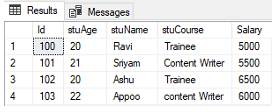Example-

COUNT()-     The following statement is to find the number of row in a column of ‘TeacherDetails’ table.

select count(stuCourse) from TeacherData;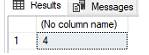AVG()-        The following statement is to find the Average of numerical column values of ‘TeacherDetails’ table.

select avg(Salary) from TeacherData;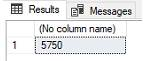SUM()-     The following statement is to find the sum of the numerical column values in table ‘TeacherDetails’.

select sum(Salary) from TeacherData;SQL MIN and MAX –

The SQL function MIN() and MAX() is to find the minimum and maximum values form database table.

The MIN() function is used to find the minimum value from table.

The MAX() function is used to find the maximum value from table.

MIN( )      Syntax-

SELECT MIN(column_name) FROM table_name WHERE condition;

MAX() Syntax-

SELECT MAX(column_name) FROM table_name WHERE condition; MAX() function

Example-

-                   Let have a table ‘TeacherDetails’.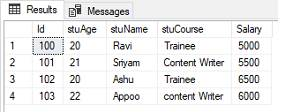MAX()-    Follow the statement to find the maximum value from ‘TeacherDetails’ table.

select max(Salary) from TeacherData;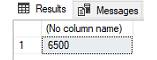To find the record which has maximum Salary then you can use the following statement.

select * from TeacherData where Salary=(select max(Salary) from TeacherData);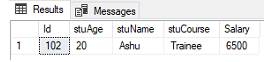MIN()-        The following statement is used to find the minimum Salary from ‘TeacherDetails’ table.

select min(Salary) from TeacherData;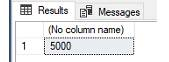To find the record of that row which has minimum value is like as-

select * from TeacherData where Salary =(select min(Salary) from TeacherData);